Multiplication of fractionsCustom SearchMULTIPLICATION The fact that multiplication by a fraction does not increase the value of the product may confuse those who remember the definition of multiplication presented earlier for whole numbers. It was stated that 4(5) means 5 is taken as an addend 4 times. How is it then that .1/2(4) is 2, a number less than 4? Obviously our Idea of multiplication must be broadened. Consider the following products: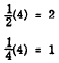Notice that as the multiplier decreases, the product decreases, until, when the multiplier is a fraction, the product is less than 4 and continues to decrease as the fraction decreases. The fraction introduces the "part of" idea: 1/2(4) means 1/2 of 4; 1/4(4) means 1/4 of 4.  The definition of multiplication stated for whole numbers may be extended to include fractions. Since 4(5) means that 5 is to be used 4 times as an addend, we can say that with fractions the numerator of the multiplier tells how many times the numerator of the multiplicand is to be used as an addend. By the same reasoning, the denominator of the multiplier tells how many times the denominator of the multiplicand is to be used as an addend. The following examples illustrate the use of this idea: 1. The fraction 1/12 is multiplied by the whole number 4 as follows: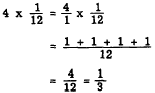This example shows that 4 (1/12) is the same as 4(1)/12. Another way of thinking about the multiplication of l/12 by 4 is as follows:2. The fraction 2/3 is multiplied by l/2 as follows: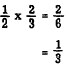member that we can simplify division by showing both dividend and divisor as the indicated From these examples a general rule is developed: To find the product of two or more fractions multiply their numerators together and write the result as the numerator of the product; multiply their denominators and write the result as the denominator of the product; reduce the answer to lowest terms. In using this rule with whole numbers, write each whole number as a fraction with 1 as the denominator. For example, multiply 4 times 1/12 as follows: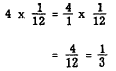In using this rule with mixed numbers, rewrite all mixed numbers as improper fractions before applying the rule, as follows: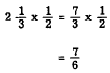A second method of multiplying mixed numbers makes use of the distributive law. This law states that a multiplier applied to a two-part expression is distributed over both parts. For example, to multiply 6 1/3 by 4 we may rewrite 6 1/3 as 6 + l/3. Then the problem can be written as 4(6 + 1/3) and the multiplication proceeds as follows: 4(6 + 1/3) = 24 + 4/3     = 25 + l/3     = 25 1/3 Cancellation Computation can be considerably reduced by dividing out (CANCELING) factors common to both the numerator and the denominator. We recognize a fraction as an indicated division. Thinking of 6/9 as an indicated division, we remember that we can simplify division by showing both dividend and divisor as the indicated products of their factors and then dividing like factors, or canceling. Thus,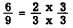Dividing the factor 3 in the numerator by 3 in the denominator gives the following simplified result:This method is most advantageous when done before any other computation. Consider the example,The product in factored form isRather than doing the multiplying and then reducing the result 6/30 it is simpler to cancel like factors first, as follows: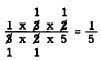Likewise,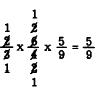Here we mentally factor 6 to the form 3 x 2, and 4 to the form 2 x 2. Cancellation is a valuable tool in shortening operations with fractions. The general rule may be applied to mixed numbers by simply changing them to improper fractions. Thus,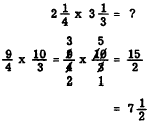Practice problems. Determine the following products, using the general rule and canceling where possible: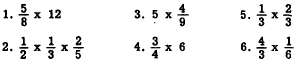Answers:The following problem illustrates the multiplication of fractions in a practical situation. EXAMPLE: Find the distance between the center lines of the first and fifth rivets connecting the two metal plates shown in figure 4-7 (A). SOLUTION: The distance between two adjacent rivets, centerline to centerline, is 4 1/2 times the diameter of one of them. Thus,There are 4 such spaces between the first and fifth rivets. Therefore, the total distance, D, is found as follows: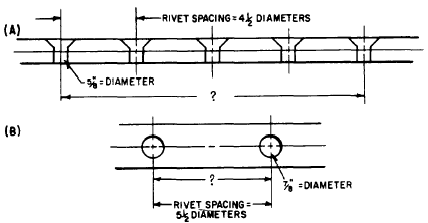Figure 4-7.-Application of multiplication of fractions in determining rivet spacing. The distance is 11 a inches Practice problem. Find the distance between the centers of the two rivets shown in figure 4-7 (B). Answer: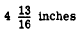Integrated Publishing, Inc. - A (SDVOSB) Service Disabled Veteran Owned Small Business